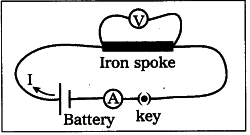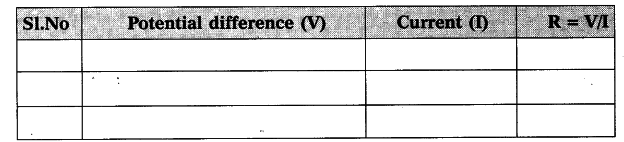# State Ohm's law

State Ohm’s law. Suggest an experiment to verify it and explain the procedure.

Ohm’s Law :
The current through a cortductor element is proportional to the potential difference applied between the ends, provided the temperature remains constant.
V = IR
Aim : To show that the ratio V/I is a constant for a conductor.
Materials required : 5 dry cells of 1.5V each, conducting wires, an ammeter, a voltmeter, thin iron spoke of length 10cm, LED and key.
Procedure : Connect a circuit as shown in figure. Solder the conducting wires to the ends of the iron spoke. Close the key. Note the readings of current from ammeter and potential difference from voltmeter in table.i) Connect a circuit as shown in the figure.
ii) Solder the conducting wires to the ends of the iron spoke and close the key.
iii) Note the reading of ammeter (current) and voltmeter (potential difference) and tabulate them.
iv) Now connect two cells in the circuit and note the respective readings of am-meter and voltmeter in the above table.
v) Repeat the above procedure using three cells and, cells and five cells respec-tively.
vi) Record the values of potential difference (V) and current (I) corresponding to each case in the above table.
vii) Find V/I for each set of values.
viii) We notice that V/I is constant.
V∝ I
ix) From this we can conclude that potential difference between the ends of the iron spoke is directly proportional to the current passing through it. This is Ohm’s law.